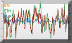## Returns

Compare two timeserie returns during the day.

Create a function `ts` asking for trade price for a stock for a day.

Create another function `tsreturn` computing return by dividing by open value and substarcting 1.See in playground

``````(defn ts[code date]
(timeserie @trade-price "trth" code date)
)
(defn tsreturn[code date]
(- (/ (ts code date) (vget (ts code date) 0 )) 1)
)
[
(tsreturn "BNPP.PA" 2016-02-01)
(tsreturn "SOGN.PA" 2016-02-01)
]
``````

## VWAP

Compute Volum Weighted Average Price since beginning of the day by creating `vwap` function. Graph versus trade prices.

Use graphsample to get same graph but with less data transmission.See in playground

``````(defn vwap [source code date]
(/
(sigma
(*
(timeserie @trade-price source code date)
(timeserie @trade-volume source code date)
)
)
(sigma
(timeserie @trade-volume source code date)
)
)
)
[
(graphsample 1920 (timeserie @trade-price "bitstamp" "BTC" 2018-02-17))
(graphsample 1920 (vwap "bitstamp" "BTC" 2018-02-17))
]
``````

## TWAP

Compute Time-Weighted Average Price on a 10 minutes sliding window by creating `twap` function.

Use graphsample to get same graphical representation but with less data transmission.See in playground

``````;time weighted over w
(defn twap[w ts]
(/
(sliding w sum (* (forward ts) (delta (time-as-value ts))))
(sliding w sum (delta (time-as-value ts)))
)
)
[
(timeserie @trade-price "bitstamp" "BTC" 2018-02-16)
(twap
10m
(timeserie @trade-price "bitstamp" "BTC" 2018-02-16)
)
(twap
1h
(timeserie @trade-price "bitstamp" "BTC" 2018-02-16)
)
]
``````

## Hayashi Yoshida

Compute Hayashi Yoshida correlation estimator over a slinding window.

Here is for example how Hayashi Yoshida could be implemented directly in LispTick. To have a nicer input we create a midserie function computing mid from bid and ask, keeping values in right time range.

We also compare result to trade price correlation which seems to be way more signifiant.

Use graphsample to get same graphical representation but with less data transmission.See in playground

``````(def start T09:10) (def stop T17:30)
(defn midserie [code date]
(prune
(* 0.5
(+
(restrict (timeserie @bid-price "trth" code date) start stop)
(restrict (timeserie @ask-price "trth" code date) start stop)
)
)
)
)
(defn hayashi-yoshida [w x y]
(/
(sliding w sum
(*
(delta x)
(delta y)))
(sqrt
(*
(sliding w sum (* (delta x) (delta x)))
(sliding w sum (* (delta y) (delta y)))
)
)
)
)
[
(label "1h mid correl"
(graphsample 1920
(hayashi-yoshida
1h
(midserie "BNPP.PA" 2016-02-01)
(midserie "SOGN.PA" 2016-02-01)
)
)
)
(label "1h trade correl"
(graphsample 1920
(hayashi-yoshida
1h
(prune (timeserie @trade-price "trth" "BNPP.PA" 2016-02-01))
(prune (timeserie @trade-price "trth" "SOGN.PA" 2016-02-01))
)
)
)
]
``````

## Finance algorithms applied to hive monitoring

Applying finance time series algorithms to hive temperature allows bees health monitoring.

When bees are sick, they are unable to keep inside hive temperature constant. So if inside temperature is strongly correlated to outside temperature bees are probably sick.

Here we are using Hayashi-Yoshida to compute correlation between in and out T° as they are asynchronous time series.

Then we smooth result using time-weighted average. If result is bigger than a defined threshold we switch alert ON.

Data gracefully provided by MellispheraSee in playground

``````;correlation over w
(defn hayashi-yoshida [w x y]
(/
(sliding w sum
(*
(delta x)
(delta y)))
(sqrt
(*
(sliding w sum (* (delta x) (delta x)))
(sliding w sum (* (delta y) (delta y)))
)
)
)
)
;time weighted over w
(defn twap[w ts]
(/
(sliding w sum (* (forward ts) (delta (time-as-value ts))))
(sliding w sum (delta (time-as-value ts)))
)
)
;alert when weighted average correlation >threshold
(defn alert[in out whayashi wtwap th]
(sign (- (twap wtwap (hayashi-yoshida whayashi in out)) th))
)
;parameters definition
(def start 2018-05-12)
(def stop 2018-07-07)
(def out
(timeserie @Temp_ext "dataview" "Ruche000" start stop)
)
(def in
(timeserie @Temp_int "dataview" "Ruche000" start stop)
)
(defn norm[ts]
(* 5 (+ 1 ts))
)
(def w1 12h)
(def w2 6h)
(def threshold 0.83)
[
(label "T°hive" in)
(label "Ok" (norm (* -1 (alert in out w1 w2 threshold))))
(label "T°out" out)
(label "Alert" (norm (alert in out w1 w2 threshold)))
(label "Raw correlation" (hayashi-yoshida w1 in out))
(label "Smoothed correlation" (twap w2 (hayashi-yoshida w1 in out)))
]
``````